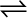7.10 At 450K, Kp= 2.0 × 1010/bar for the given reaction at equilibrium.

2SO2(g) + O2(g)2SO3 (g)

What is Kc at this temperature?

For the given reaction,
$∆\mathrm{n}=2-3=-1$

${\mathrm{K}}_{\mathrm{p}}={\mathrm{K}}_{\mathrm{c}}\left(\mathrm{RT}\right)∆\mathrm{n}$